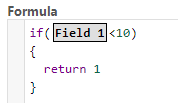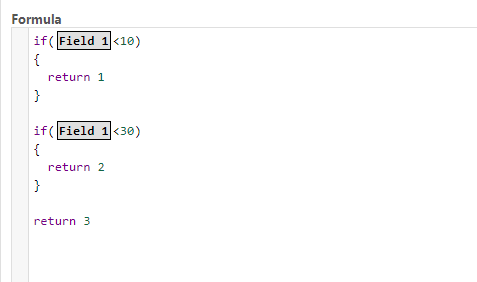# Blog

Posted by:

You are here:
• Conditions

## Introduction

You can create conditions using an structure like this

``````if(comparison)
{
return value
}
``````

or if you prefer you can use a shorter structure like this:

``````if(comparison)
value``````

Replace “comparison” with the comparison you want and “value” with the value that need to be used, example:In this condition if field 1 has a value less than 10 the value ‘1’ will be used by this formula

You can use multiple if conditions in the formula, like in this exampleIf Field 1 is less than 10 the formula will use ‘1’ if Field 1 is less than 30 it will use 2, otherwise it will use 3

## Type of comparissons

You can use these comparisons

 Comparison Name Example > Greater than if(10>20) >= Greater or equal than if(10>=20) < Less than if(10<20) <= Less or equal than if(10<=20) == Equal to if(10==20) != Not equal to if(10!=20) contains Contains if(“Hello word” contains “word”) not contains Not Contains if(“Hello word” not contains “word”)